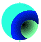##Hands On Physics

### The Great Bungee Jump Core Project Step 3: Calibrating the TimerOverview

This page shows how to get your timer working accurately. The idea is to time intervals you already know. By fine-tuning your timer, you can get it to accurately predict these values you know. Then it should be very accurate under similar conditions used in the Bungee Jump.

### Computing the time

We will use freely falling objects to generate short, known times. An object, starting from rest, requires a time, t, to fall a distance, d, in free fall under the influence of gravity, g. The realtion between these variables is

d = (1/2)*g*t^2

In this equation, t^2 means "t squared". To understand where this equation comes from, see the math concepts page.

This equation can be solved for t, to give

t = sqrt(2*d / g)

In this equation, sqrt(...) means "take the square root of everything inside the parentheses". This equation tells you how long it takes for an object to drop from rest through a distance, d.

In the SI system of measurement distance is measured in meters, acceleration in meters per second squared, and time is measured in seconds. To sufficient accuracy, g is 9.81 m/s^2.

### Calibrating the Timer

Your timing circuit reports voltage (actually, millivolts). Your task is to relate these voltage readings to time. For times much less than the product R*C, the voltage is proporational to the time. This can be written as

t = k*V

where k is some constant. Your goal is to figure out what k is. You can think of it as a "fudge factor" because it is the number needed to make V read in seconds. The fancy word for this is a "calibration constant".

One way to compute k is to plot t as a function of V, using different times. You should get a straight line through the origin and its slope will be k. You measure V with the voltmeter and compute t from d. So this means you have to do a series of measurements for different distances, d, of fall.

From the mathematics of the circuit, we know what k should be:

k = R*C/Vo

Here Vo is the voltage applied to the circuit, R is the value of the resistor, and C is the value of the capacitor. It is hard to measure C accurately. If you have the instruments to measure C, you can just compute k. Go ahead with the experiment anyway to see whether these two different ways of measuring k give the same result.

## The Experiment

1. Set up the experiment. Use your tower and timing circuit. Measure the power supply voltage Vo. If the voltage is accidentally changed, you need to be able to re-set it exactly, otherwise you will have to recalibrate your timer at the new voltage.

2. Take a first measurement. Move switch 2 near the bottom of the tower. Measure the distance, d, from the mass when it is held in clothespin to switch 2. Think about exactly what points on the mass and switch to measure between. Drop the mass several times until you get consistent voltage readings. Average your good readings.

3. Repeat your measurement. You want ten measurements for approximately evenly-spaced times. Figure out what distances you need for this. Repeat the measurement process for each height. Record your data in a table.

4. Analyze your data. Make a graph of the data showing time of fall as a function of millivolts. Draw a best-fit straight line through your data. Compute k.

## Challenges

• Is the plot on your graph a straight line? Does is go through the origin?

• If your plot is a straight line, what does this tell you about the function of your circuit as a timer? What if the plot is not a straight line?

• What is the maximum number of seconds that can be timed with your circuit? How far would the weight fall in that time?

• Using k, determine the value of the capacitor in your circuit.

• Is the velocity of the falling object constant, or does it change? Determine the acceleration from your data. Describe how you do this.

## Reporting

Report your data and graph. You should also make a sketch of the falling object and identify the forces on it using vectors.

Previous Page || Up a Level || Index || Next Page